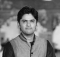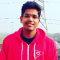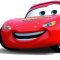## Difference Between Prim’s and Kruskal’s AlgorithmUpdated on 02-Mar-2021 05:02:09
In this post, we will understand the differences between Prim's and Kruskal's algorithms.Kruskal's algorithm for Mininum Spanning Tree (MST)When a connected and undirected graph is given, a spanning tree of such a graph is the subgraph which is a tree that connects all of the vertices.A single graph can have multiple spanning trees.A minimum spanning tree (MST) (also known as minimum weight spanning tree) for a weighted, connected and undirected graph is a spanning tree that weighs less than or equal to the weight of every other spanning tree.The weight of a spanning tree is determined by adding the weights ... Read More

## Yen's k-Shortest Path Algorithm in Data StructureUpdated on 23-Feb-2021 06:35:29
Instead of giving a single shortest path, Yen’s k-shortest path algorithm gives k shortest paths so that we can get the second shortest path and the third shortest path and so on.Let us consider a scenario that we have to travel from place A to place B and there are multiple routes available between place A and place B, but we have to find the shortest path and neglect all the paths that are less considered in terms of its time complexity in order to reach the destination.Let us understand with an example-Consider the given example as the bridge which ... Read More

## Algorithm to construct an Expression Tree in Data StructureUpdated on 23-Feb-2021 18:11:51
Expression treesExpression trees are those in which the leaf nodes have the values to be operated, and internal nodes contain the operator on which the leaf node will be performed.Example4 + ((7 + 9) * 2) will have an expression tree as followsAlgorithm to Construct an Expression TreeLet T be the expression tree.If T is not NULL:   If T->data is an operand:      return T.data   A = solve(T.left)   B = solve(T.right)   --> Calculate operator for 'T.data' on A and B, and call recursively,       return calculate(A, B, T.data)How to construct an expression tree?To construct an Expression Tree for ... Read More

## Insertion in the Red Black Tree in Data StructureUpdated on 05-Feb-2021 12:42:32
Red Black Tree is a Self-Balanced Binary Search Tree in which each node of the tree is colored with either Red or Black. There are three types of operations we can perform on a Red Black Tree – Searching, Insertion and Deletion.Let us suppose we have to insert an element in the following Red Black Tree.To insert an element in a red-black tree the idea is very simple − we perform insertion just like we insert in a regular binary tree. We start off from the root node by checking the color of the node and insert it into a ... Read More

## Floyd Cycle Detection Algorithm to detect the cycle in a linear Data StructureUpdated on 05-Feb-2021 12:22:42
Floyd Cycle is one of the cycle detection algorithms to detect the cycle in a given singly linked list.In the Floyd Cycle algorithm, we have two pointers that initially point at the head. In Hare and Tortoise’s story, Hare moves twice as fast as Tortoise, and whenever the hare reaches the end of the path, the tortoise reaches the middle of the path.AlgorithmInitialize Hare and Tortoise at the head node of the List.Initially, the hare moves twice as fast as the tortoise.Move the hare and tortoise both and find if the hare reaches the end of the Linked List, return ... Read More

## Auto-complete feature using TrieUpdated on 21-Sep-2020 13:19:12
We have a Trie, and when a user enters a character, we have to show the matching string the Trie. This feature we call it as auto-completion. For example, if a Trie contains "xyzzzz, ""xyz, " "xxxyyxzzz" and when the user enter xy, then we have to show them xyzzzz, xyz, etc.., Steps to achieve the result.Search for the string using the standard Trie algorithm.If the string is not present, then return -1.If the string is present and is the end of a word in Trie, then print the string.If the matching string doesn't have any node, then return.Else print ... Read More

## Segment Trees in Data StructureUpdated on 11-Aug-2020 07:52:15
In this section we will see what is the segment tree. Before discussing that, let us see one problem.Suppose we have an array arr[0, …, n-1], We can do following operations −Find the sum of elements from index l to r, where 0 ≤ l ≤ r ≤ n-1Change the value of a specified element of the array to a new value x. We need to do arr[i] = x. The i in range 0 to n – 1.We can solve this problem by using the Segment tree. The segment tree can help us to get the sum and query ... Read More

## Interval Trees in Data StructureUpdated on 11-Aug-2020 07:50:46
In this section we will see what is the interval tree. As the name suggests, that the interval trees are the trees which are associated with the intervals. So before discussing about the interval trees, let us see the elementary intervals.An interval is basically a range. So if one interval is written as [a, b] it indicates that the range is starting from a, and ending at b.Now suppose there is an interval [10, 20]. So there are three range values. First one is -∞ to 10, 10 to 20 and finally 20 to ∞Now, suppose we will create second ... Read More

## B+ tree Deletion in Data StructureUpdated on 11-Aug-2020 07:47:36
Here we will see, how to perform the deletion of a node from B+ Tree. Suppose we have a B+ Tree like below 7minus;Example of B+ Tree −Deletion has two parts. At first we have to find the element. That strategy is like the querying. Now for deletion, we have to care about some rules. One node must have at-least m/2 elements. So if we delete, one element, and it has less than m-1 elements remaining, then it will adjust itself. If the entire node is deleted, then its children will be merged, and if their size is same as ... Read More

## B+ tree Insertion in Data StructureUpdated on 11-Aug-2020 07:42:05
Here we will see, how to perform the insertion into a B+ Tree. Suppose we have a B+ Tree like below −Example of B+ Tree −To insert an element, the idea is very similar to the B-Tree, if one element is inserted, that will be stored at the leaf node. If that is present in some internal node, then it will also be there, at the leaf as right child of itself.Suppose we want to insert 65 into the tree. So that is greater than 60, and less than 75. Then it will be inserted into the middle sub-tree. Now, ... Read More